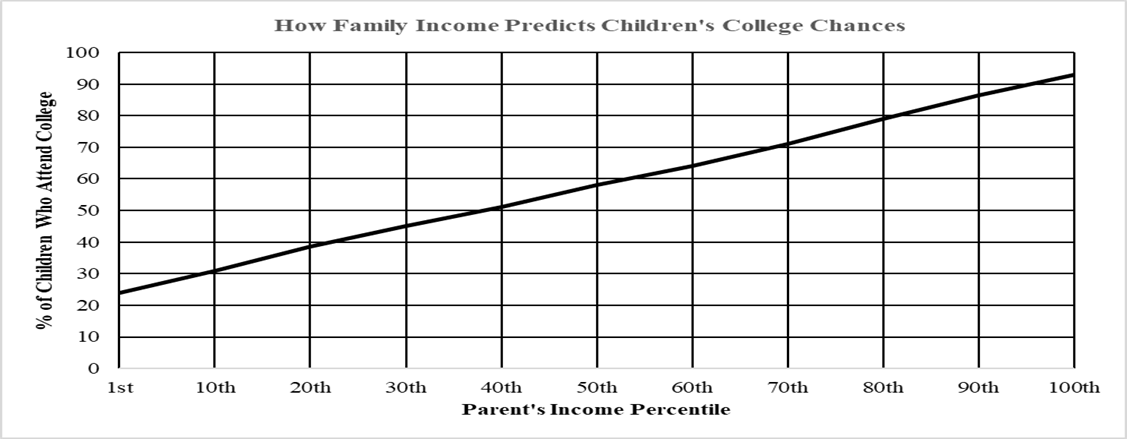# How Family Income Predicts Children's College Chances100SSO7060504O30201020th60th7oth50thParent's Income Percentile1st10th30th40th80th90th10Oth

Question
224 views

start answering these questions based on the graph attached. Thanks
1.) Estimate the equation of the line.

2.) Use the slope of the line in a meaningful sentence or two.

3.) What observations can you interpret from the graph?

4.) A recent survey found that in a particular city of all poor children born in 1980, 11,500 enrolled in college, and 1024 graduated from college by age 24. Scale this rate to 100.

5.) From the information in the article, in a town consisting of 2,300 rich students born in 1980, how many would you expect to graduate college by the age of 24? Use the 95th percent income percentile as constituting rich (note: what % of children attend college based upon the parents 95th income percentile). Scale this rate to 100.

6.) From a random sample: Out 1,000 rich kids born in 1980, how many will attend college and how many will graduate from college by the age of 24?help_outlineImage TranscriptioncloseHow Family Income Predicts Children's College Chances 100 SSO 70 60 50 4O 30 20 10 20th 60th 7oth 50th Parent's Income Percentile 1st 10th 30th 40th 80th 90th 10Oth fullscreen
check_circle

Step 1

Hello there! I see that there are more number of questions on this graph. I can only help with first 3 questions in it. According to our policies cannot answer more than that. Kindly make a new request for remaining questions.

Given a graph with Parents Income percentile on x axis and percentage of children who attend college on y axis.

Here we choose two data points (10,30) and (30,45) as two data points on the line and we use them to find the line equation.

Step 2

The line equation of a straight line passing through (x1,y1) and (x2,y2) is given as shown below

Substituting the two known data points on line (10,30) and (30,45) we get the line equation as  x -2y = 50

Step 3

Let us write the equation of line in slope intercept form y=mx+b where m is slope and b is y intercept as shown below

y = (1/2)x + 25.

From this we can say that the slope of the line is 1/2.

As the slope is positive we can say that if the percentile of parents income...

### Want to see the full answer?

See Solution

#### Want to see this answer and more?

Solutions are written by subject experts who are available 24/7. Questions are typically answered within 1 hour.*

See Solution
*Response times may vary by subject and question.
Tagged in

### Probability